# A chain of departmental stores has outlets in Delhi, Mumbai - CAT DILR questions – Data Interpretation

## CAT 2020 - Slot 2 - Question 3 - A chain of departmental stores has outlets in Delhi, Mumbai

### A chain of departmental stores has outlets in Delhi, Mumbai, Bengaluru and Kolkata. The sales are categorized by its three departments – ‘Apparel’, ‘Electronics’, and ‘HomeDecor’. An Accountant has been asked to prepare a summary of the 2018 and 2019 sales amounts for an internal report. He has collated partial information and prepared the following table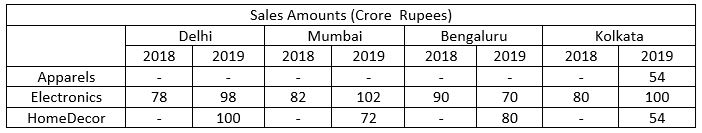The following additional information is known. 1. The sales amounts in the Apparel departments were the same for Delhi and Kolkata in 2018. 2. The sales amounts in the Apparel departments were the same for Mumbai and Bengaluru in 2018. This sales amount matched the sales amount in the Apparel department for Delhi in 2019. 3. The sales amounts in the HomeDecor departments were the same for Mumbai and Kolkata in 2018. 4. The sum of the sales amounts of four Electronics departments increased by the same amount as the sum of the sales amounts of four Apparel departments from 2018 to 2019. 5. The total sales amounts of the four HomeDecor departments increased by Rs 70 Crores from 2018 to 2019. 6. The sales amounts in the HomeDecor departments of Delhi and Bengaluru each increased by Rs 20 Crores from 2018 to 2019. 7. The sales amounts in the Apparel departments of Delhi and Bengaluru each increased by the same amount in 2019 from 2018. The sales amounts in the Apparel departments of Mumbai and Kolkata also each increased by the same amount in 2019 from 2018. 8. The sales amounts in the Apparel departments of Delhi, Kolkata and Bengaluru in 2019 followed an Arithmetic Progression.

Q9. In HomeDecor departments of which cities were the sales amounts the highest in 2018 and 2019, respectively?

a. Delhi and Delhi

b. Mumbai and Delhi

c. Mumbai and Mumbai

d. Bengaluru and Delhi

9. A.

Before we begin solving, let’s try to apply the easiest points to the table to see how much info we can get (Key is to apply the easiest looking rules first so that we can get as many missing numbers as possible, not a good idea to apply the rules in the order that they are given)

For now, let us apply points 1, 2, 3, 6 and see how the table looks: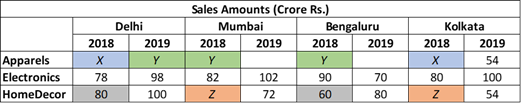Now let’s apply point 5 so that we can get the missing value Z

(100+72+80+54) – (80 + Z + 60 + Z) = 70

306 – 140 – 2(Z) = 70

166 – 2(Z) = 70

2(Z) = 96

Z = 48

This is how the table looks as of now: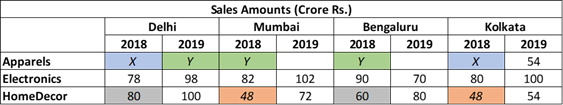From the available info, we can get the answer to this question. Delhi had the highest sales in 2018 and 2019.

Let’s continue to solve the table, let’s apply point 7 now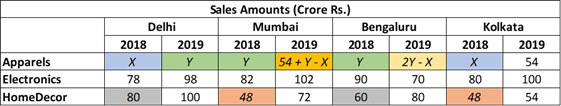Let’s apply point 8.

Since Sales for Delhi, Kolkata and Bangalore in 2019 form an AP, the following must be true:

K (2019) – D (2019) = B (2019) – K (2019)

54 – Y = (2Y – X) – 54

108 = 3Y – X ………………………(Equation 1)

Finally, let’s apply point 4.

(98 + 102 + 70 + 100) – (78 + 82 + 90 + 80) = (Y + 54 + Y – X + 2Y – X + 54) – (X + Y + Y + X)

370 – 330 = (108 + 4Y – 2X) – (2Y + 2X)

40 = 108 + 2Y – 4X

4X – 2Y = 68………………………(Equation 2)

Solve equations 1 and 2

4X – 2Y= 68

12Y – 4X = 432

10Y = 500

Y = 50

X = 42

The finished table looks like this: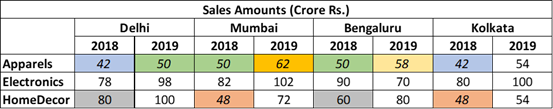Q10. What was the increase in sales amount, in Crore Rupees, in the Apparel department of Mumbai from 2018 to 2019?

a. 12

b. 10

c. 5

d. 8

10. A.

Before we begin solving, let’s try to apply the easiest points to the table to see how much info we can get (Key is to apply the easiest looking rules first so that we can get as many missing numbers as possible, not a good idea to apply the rules in the order that they are given)

For now, let us apply points 1, 2, 3, 6 and see how the table looks: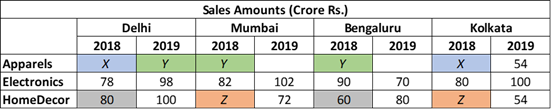Now let’s apply point 5 so that we can get the missing value Z

(100+72+80+54) – (80 + Z + 60 + Z) = 70

306 – 140 – 2(Z) = 70

166 – 2(Z) = 70

2(Z) = 96

Z = 48

This is how the table looks as of now: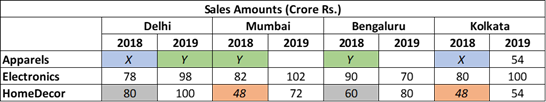Let’s continue to solve the table, let’s apply point 7 now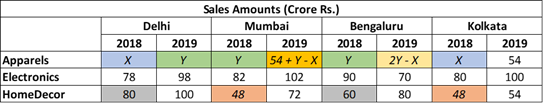Let’s apply point 8.

Since Sales for Delhi, Kolkata and Bangalore in 2019 form an AP, the following must be true:

K (2019) – D (2019) = B (2019) – K (2019)

54 – Y = (2Y – X) – 54

108 = 3Y – X ………………………(Equation 1)

Finally, let’s apply point 4.

(98 + 102 + 70 + 100) – (78 + 82 + 90 + 80) = (Y + 54 + Y – X + 2Y – X + 54) – (X + Y + Y + X)

370 – 330 = (108 + 4Y – 2X) – (2Y + 2X)

40 = 108 + 2Y – 4X

4X – 2Y = 68………………………(Equation 2)

Solve equations 1 and 2

4X – 2Y= 68

12Y – 4X = 432

10Y = 500

Y = 50

X = 42

The finished table looks like this: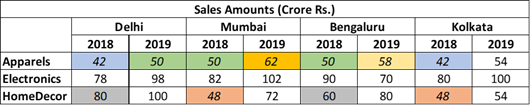With the finished table the increase in sales amount, in Crore Rupees, in the Apparel department of Mumbai from 2018 to 2019 can be found out to be 12.

Q11. Among all the 12 departments (i.e., the 3 departments in each of the 4 cities), what was the maximum percentage increase in sales amount from 2018 to 2019?

a. 75

b. 50

c. 25

d. 28

11. B.

Before we begin solving, let’s try to apply the easiest points to the table to see how much info we can get (Key is to apply the easiest looking rules first so that we can get as many missing numbers as possible, not a good idea to apply the rules in the order that they are given)

For now, let us apply points 1, 2, 3, 6 and see how the table looks: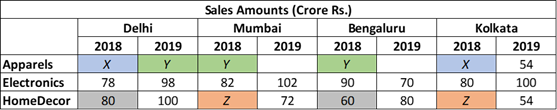Now let’s apply point 5 so that we can get the missing value Z

(100+72+80+54) – (80 + Z + 60 + Z) = 70

306 – 140 – 2(Z) = 70

166 – 2(Z) = 70

2(Z) = 96

Z = 48

This is how the table looks as of now: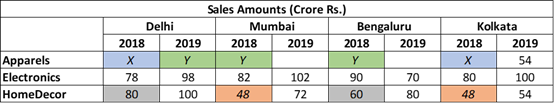Let’s continue to solve the table, let’s apply point 7 now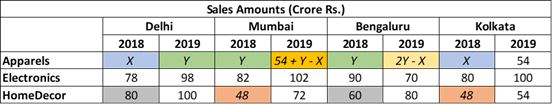Let’s apply point 8.
Since Sales for Delhi, Kolkata and Bangalore in 2019 form an AP, the following must be true:

K (2019) – D (2019) = B (2019) – K (2019)
54 – Y = (2Y – X) – 54
108 = 3Y – X ………………………(Equation 1)

Finally, let’s apply point 4.

(98 + 102 + 70 + 100) – (78 + 82 + 90 + 80) = (Y + 54 + Y – X + 2Y – X + 54) – (X + Y + Y + X)
370 – 330 = (108 + 4Y – 2X) – (2Y + 2X)
40 = 108 + 2Y – 4X
4X – 2Y = 68………………………(Equation 2)

Solve equations 1 and 2

4X – 2Y= 68
12Y – 4X = 432
10Y = 500

Y = 50
X = 42

The finished table looks like this: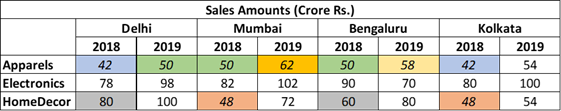A quick glance at the table can tell us that the % will be highest where the base is low and the increase is high. A strong candidate is Mumbai in HomeDecor from 2018-19 (50% increase), no other cell seems to fit the bill

Q12. What was the total sales amount, in Crore Rupees, in 2019 for the chain of departmental stores?

a. 900

b. 150

c. 750

d. 600

12. A.

Before we begin solving, let’s try to apply the easiest points to the table to see how much info we can get (Key is to apply the easiest looking rules first so that we can get as many missing numbers as possible, not a good idea to apply the rules in the order that they are given)

For now, let us apply points 1, 2, 3, 6 and see how the table looks: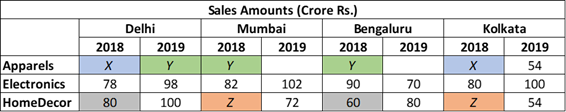Now let’s apply point 5 so that we can get the missing value Z
(100+72+80+54) – (80 + Z + 60 + Z) = 70
306 – 140 – 2(Z) = 70
166 – 2(Z) = 70
2(Z) = 96
Z = 48

This is how the table looks as of now: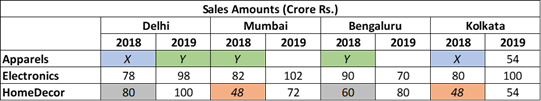Let’s continue to solve the table, let’s apply point 7 now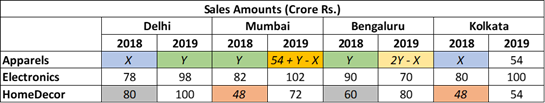Let’s apply point 8.

Since Sales for Delhi, Kolkata and Bangalore in 2019 form an AP, the following must be true:

K (2019) – D (2019) = B (2019) – K (2019)

54 – Y = (2Y – X) – 54

108 = 3Y – X ………………………(Equation 1)

Finally, let’s apply point 4.

(98 + 102 + 70 + 100) – (78 + 82 + 90 + 80) = (Y + 54 + Y – X + 2Y – X + 54) – (X + Y + Y + X)

370 – 330 = (108 + 4Y – 2X) – (2Y + 2X)

40 = 108 + 2Y – 4X

4X – 2Y = 68………………………(Equation 2)

Solve equations 1 and 2

4X – 2Y= 68

12Y – 4X = 432

10Y = 500

Y = 50

X = 42

The finished table looks like this: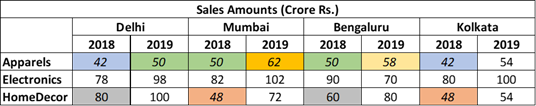By adding, using the finished table, we arrive at the answer 900.

### Past Year Question Paper & SolutionsCounselling Session
By IIM Mentor

#### Free Material Area